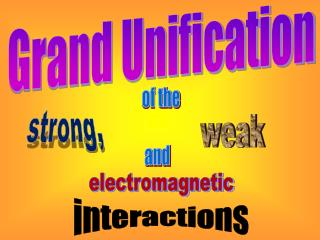# Grand Unification - PowerPoint PPT PresentationDownload PresentationGrand Unification

Grand UnificationDownload Presentation## Grand Unification

- - - - - - - - - - - - - - - - - - - - - - - - - - - E N D - - - - - - - - - - - - - - - - - - - - - - - - - - -
##### Presentation Transcript

1. - Grand Unification of the strong, weak and electromagnetic interactions

2. Standard Model SU(3) x SU(2) x U(1)

3. problems: three unrelated coupling constants? quantization of electric charges? solutions? =>

4. SU(3) x SU(2) x U(1) subgroup of a semisimple group

5. Leptons as a fourth color ( Pati-Salam - 1974 ) SU(3) x SU(2) x U(1) < SU (4) x SU (2, L) x SU (2, R)

6. ( ( SU(4)

7. (f, L): (4, 2, 1) (f, R): (4, 1, 2)

8. ( ( =(4,1,2) R requires: righthanded partner of SM neutrino + new "righthanded" W-bosons

9. SU(4) ==> SU(3) x (B-L)

10. - SU(3) x SU(2) x U(1) SU(5) Georgi – Glashow 1974

11. - SU(3) x SU(2) x U(1) SO(10) Fritzsch – Minkowski Georgi 1975

12. rank of a group: number of diagonal elements rank U(1) = 1 rank SU(n)=n-1 rank SU(2)=1 rank SU(3)=2 rank SU(3) x SU(2) x U(1) =4 rank SU(5) = 4

13. ===> SU(5) is smallest group to embed the Standard Model

14. SU(5) - theory: SU(3) x SU(2) x U(1) < SU(5) fermions of one family in two different representations:

15. 5-represenation: 10-represenation: (antisymmetric second rank tensor)

16. sum of electric charges = 0 => charge quantization

17. sum of electric charges = 0

18. - quarksandleptons in the same multiplet => decayoftheproton intoleptons ??? (later)

19. SU(5) ==> SU(3) x SU(2) x U(1) - u d u d neutrino positron - electron -

20. one family of leptons and quarks

21. Gauge bosons in SU(5) > SU(3) x SU(2) x U(1):adjoint representation ~ (24)5 x 5* = 24 + 124 = (8,1) + (1,3) + (1,1) + (3,2) + (3*,2)

22. - 24 = 12 gauge bosons: 8 gluons, 2 W-bosons, one Z boson, one photon + 12 superheavy color triplet gauge bosons

23. Interesting features of SU(5): 1. electric charge => quantized (charge operator is generator of a simple group)

24. 2. Electric charges of quarks are related to charge of electron

25. Grand unification takes placeabove a very high unified mass scale M. There is only one unified coupling constant g. The coupling constants for QCD and for the SU(2) x U(1) theory are the same, apart from Clebsch-Gordan coefficients.

26. The different values of the three coupling constants at low energies are due to renormalization effects.

27. -

28. -

29. - convergence of the coupling constants - grand unification -

30. - "Higgs" particles in the adjoint representation (24) H: 5 x 5 hermitian traceless matrix

31. SU(5) H SU(3) x SU(2) x U(1) doublet SU(3) x U(1)

32. The convergence of the three coupling constants at M implies a condition at low energies:

33. This formula is independent of the details of the grand unified theory, e.g the gauge group, but only depends on the spectrum of the fermions, described in the Standard Model.

34. F: Nr. of families

35. ==>

36. At unification energy M:

37. No convergence of coupling constants LEP data in SU(5)

38. experiment: 0.118

39. convergence of the coupling constants

40. convergence in case ofsupersymmetry energy scale of supersymmetric partners: ~ 1 TeV

41. - Each particle of the Standard Model has a supersymmetric partner: quark squark ( spin 0) lepton slepton ( spin 0) photon photino ( spin ½) gluon gluino ( spin ½) W – boson wino ( spin ½)

42. Grand unification SU(3) x SU(2) x U(1) ==>SO(10) Fritzsch - Minkowski Georgi - 1975

43. n complex numbers can be described by(2n) real numbers. SU(5) ==> SO(10)

44. SU(3) x SU(2) x U(1)< SU(4) x SU(2,L) x SU(2,R)~ SO(6) x SO(4)=> SO(10)